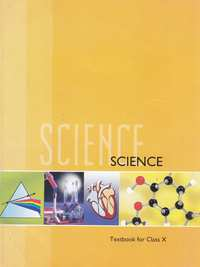# NCERT solutions for Class 10 Science chapter 11 - The Human Eye and the Colourful World [Latest edition]

#### Chapters## Chapter 11: The Human Eye and the Colourful World

[Page 198]

### NCERT solutions for Class 10 Science Chapter 11 The Human Eye and the Colourful World [Page 198]

Q 5 | Page 198

A person needs a lens of power -5.5 dioptres for correcting his distant vision. For correcting his near vision he needs a lens of power +1.5 dioptre. What is the focal length of the lens required for correcting (i) distant vision, and (ii) near vision?

Q 6 | Page 198

The far point of a myopic person is 80 cm in front of the eye. What is the nature and power of the lens required to correct the problem?

## Chapter 11: The Human Eye and the Colourful World## NCERT solutions for Class 10 Science chapter 11 - The Human Eye and the Colourful World

NCERT solutions for Class 10 Science chapter 11 (The Human Eye and the Colourful World) include all questions with solution and detail explanation. This will clear students doubts about any question and improve application skills while preparing for board exams. The detailed, step-by-step solutions will help you understand the concepts better and clear your confusions, if any. Shaalaa.com has the CBSE Class 10 Science solutions in a manner that help students grasp basic concepts better and faster.

Further, we at Shaalaa.com provide such solutions so that students can prepare for written exams. NCERT textbook solutions can be a core help for self-study and acts as a perfect self-help guidance for students.

Concepts covered in Class 10 Science chapter 11 The Human Eye and the Colourful World are Spherical Mirrors, Concave Mirror, Convex Mirror, Concave Lens, Refraction of Light, Image Formation by Convex Mirror, Sign Convention for Reflection by Spherical Mirrors, Mirror Equation/Formula, Images Formed by Spherical Mirrors, Image Formation by Concave Mirror, Reflection of Light, Law of Reflection of Light, Linear Magnification (M) Due to Spherical Mirrors, Magnification Due to Spherical Lenses, Power of a Lens, Mirrors and Its Types, Plane Mirror and Reflection, Rules for the Construction of Image Formed by a Spherical Mirror, Refraction of Light Through a Rectangular Glass Slab, Law of Refraction of Light, Refractive Index, Spherical Lens, Images Formed by Sperical Lenses, Guideline for Image Formation Due to Refraction Through a Convex and Concave Lens, Images Formed Due to Refraction Through a Concave Lens, Convex Lens, Images Formed Due to Refraction Through a Convex Lens, Sign Convention for Spherical Lenses, Lens Formula, Spherical Mirrors, Concave Mirror, Convex Mirror, Concave Lens, Refraction of Light, Image Formation by Convex Mirror, Sign Convention for Reflection by Spherical Mirrors, Mirror Equation/Formula, Images Formed by Spherical Mirrors, Image Formation by Concave Mirror, Reflection of Light, Law of Reflection of Light, Linear Magnification (M) Due to Spherical Mirrors, Magnification Due to Spherical Lenses, Power of a Lens, Mirrors and Its Types, Plane Mirror and Reflection, Rules for the Construction of Image Formed by a Spherical Mirror, Refraction of Light Through a Rectangular Glass Slab, Law of Refraction of Light, Refractive Index, Spherical Lens, Images Formed by Sperical Lenses, Guideline for Image Formation Due to Refraction Through a Convex and Concave Lens, Images Formed Due to Refraction Through a Concave Lens, Convex Lens, Images Formed Due to Refraction Through a Convex Lens, Sign Convention for Spherical Lenses, Lens Formula.

Using NCERT Class 10 solutions The Human Eye and the Colourful World exercise by students are an easy way to prepare for the exams, as they involve solutions arranged chapter-wise also page wise. The questions involved in NCERT Solutions are important questions that can be asked in the final exam. Maximum students of CBSE Class 10 prefer NCERT Textbook Solutions to score more in exam.

Get the free view of chapter 11 The Human Eye and the Colourful World Class 10 extra questions for Class 10 Science and can use Shaalaa.com to keep it handy for your exam preparation